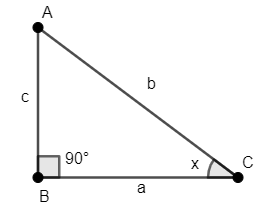QUESTION

# What is the value of ${{\sin }^{2}}x+{{\cos }^{2}}x=$____?(a) 1(b) -1(c) 0(d) $-\sqrt{2}$

Hint: Consider any right-angled triangle. Use the fact that sine of any angle is the ratio of the perpendicular to the hypotenuse and cosine of any angle is the ratio of the base to the hypotenuse. Simplify the expression using the Pythagoras Theorem of the right – angled triangle to calculate the value of the given trigonometric expression.

We have to calculate the value of ${{\sin }^{2}}x+{{\cos }^{2}}x$.
Let’s consider a right-angled triangle $\Delta ABC$, right-angled at B. Let the length of sides be $AB=c,BC=a,AC=b$ and $\angle ACB=x$ , as shown in the figure.We will use Pythagorean Theorem in the $\Delta ABC$. Thus, we have ${{a}^{2}}+{{c}^{2}}={{b}^{2}}.....\left( 1 \right)$.
We know that sine of any angle is the ratio of the perpendicular to the hypotenuse. Thus, we have $\sin \left( \angle ACB \right)=\sin x=\dfrac{c}{b}$.
Squaring the above equation on both sides, we have ${{\sin }^{2}}x=\dfrac{{{c}^{2}}}{{{b}^{2}}}.....\left( 2 \right)$.
Similarly, we know that cosine of any angle is the ratio of the base to the hypotenuse. Thus, we have $\cos \left( \angle ACB \right)=\cos x=\dfrac{a}{b}$.
Squaring the above equation on both sides, we have ${{\cos }^{2}}x=\dfrac{{{a}^{2}}}{{{b}^{2}}}.....\left( 3 \right)$.
Adding equation (2) and (3), we have ${{\sin }^{2}}x+{{\cos }^{2}}x=\dfrac{{{c}^{2}}}{{{b}^{2}}}+\dfrac{{{a}^{2}}}{{{b}^{2}}}$.
Simplifying the above equation, we have ${{\sin }^{2}}x+{{\cos }^{2}}x=\dfrac{{{c}^{2}}}{{{b}^{2}}}+\dfrac{{{a}^{2}}}{{{b}^{2}}}=\dfrac{{{c}^{2}}+{{a}^{2}}}{{{b}^{2}}}.....\left( 4 \right)$.
Substituting equation (1) in equation (4), we have ${{\sin }^{2}}x+{{\cos }^{2}}x=\dfrac{{{c}^{2}}+{{a}^{2}}}{{{b}^{2}}}=\dfrac{{{b}^{2}}}{{{b}^{2}}}=1$.
Hence, the value of ${{\sin }^{2}}x+{{\cos }^{2}}x$ is 1, which is option (a).

Note: We can also solve this question by taking the polar coordinates of a unit circle of the form $\left( \cos x,\sin x \right)$ and then substituting it in the equation of the unit circle which is ${{x}^{2}}+{{y}^{2}}=1$. One must keep in mind that ${{\sin }^{2}}x+{{\cos }^{2}}x=1$ is a trigonometric identity, i.e., it holds for all possible values of ‘x’.